MATLAB Function Referenceellipj

Jacobi elliptic functions

Syntax

• ```[SN,CN,DN]  =  ellipj(U,M)
[SN,CN,DN]  =  ellipj(U,M,tol)
```

Definition

The Jacobi elliptic functions are defined in terms of the integral:

•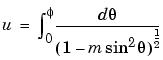Then

•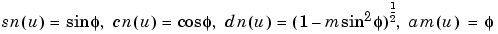Some definitions of the elliptic functions use the modulus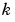instead of the parameter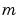. They are related by

•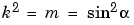The Jacobi elliptic functions obey many mathematical identities; for a good sample, see .

Description

```[SN,CN,DN] = ellipj(U,M) ``` returns the Jacobi elliptic functions `SN`, `CN`, and `DN`, evaluated for corresponding elements of argument `U` and parameter `M`. Inputs `U` and `M` must be the same size (or either can be scalar).

```[SN,CN,DN] = ellipj(U,M,tol) ``` computes the Jacobi elliptic functions to accuracy `tol`. The default is `eps`; increase this for a less accurate but more quickly computed answer.

Algorithm

`ellipj` computes the Jacobi elliptic functions using the method of the arithmetic-geometric mean . It starts with the triplet of numbers:

•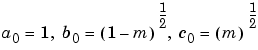`ellipj` computes successive iterates with

•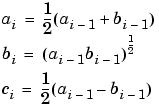Next, it calculates the amplitudes in radians using:

•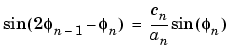being careful to unwrap the phases correctly. The Jacobian elliptic functions are then simply:

•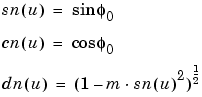Limitations

The `ellipj` function is limited to the input domain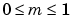. Map other values of `M` into this range using the transformations described in , equations 16.10 and 16.11. `U` is limited to real values.

See Also

`ellipke`

References

  Abramowitz, M. and I.A. Stegun, Handbook of Mathematical Functions, Dover Publications, 1965, 17.6.

© 1994-2005 The MathWorks, Inc.